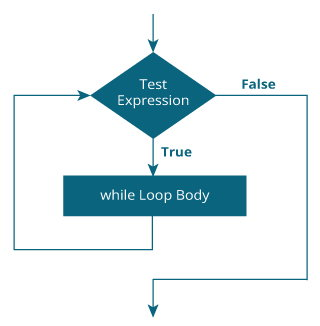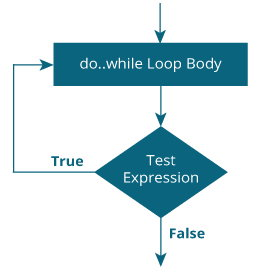C while and do...while Loop

# C while and do...while Loop

#### In this tutorial, you will learn to create while and do...while loop in C programming with the help of examples.

In programming, loops are used to repeat a block of code until a specified condition is met.

C programming has three types of loops.

1. for loop
2. while loop
3. do...while loop

In the previous tutorial, we learned about `for` loop. In this tutorial, we will learn about `while` and `do..while` loop.

## while loop

The syntax of the `while` loop is:

``````while (testExpression)
{
// statements inside the body of the loop
}``````

### How while loop works?

• The `while` loop evaluates the test expression inside the parenthesis `()`.
• If the test expression is true, statements inside the body of `while` loop are executed. Then, the test expression is evaluated again.
• The process goes on until the test expression is evaluated to false.
• If the test expression is false, the loop terminates (ends).

To learn more about test expression (when the test expression is evaluated to true and false), check out relational and logical operators.

### Flowchart of while loop### Example 1: while loop

``````// Print numbers from 1 to 5

#include <stdio.h>
int main()
{
int i = 1;

while (i <= 5)
{
printf("%d\n", i);
++i;
}

return 0;
}``````

Output

```1
2
3
4
5
```

Here, we have initialized i to 1.

1. When i is 1, the test expression `i <= 5` is true. Hence, the body of the `while` loop is executed. This prints 1 on the screen and the value of i is increased to 2.
2. Now, i is 2, the test expression `i <= 5` is again true. The body of the `while` loop is executed again. This prints 2 on the screen and the value of `i` is increased to 3.
3. This process goes on until i becomes 6. When i is 6, the test expression `i <= 5` will be false and the loop terminates.

## do...while loop

The `do..while` loop is similar to the `while` loop with one important difference. The body of `do...while` loop is executed at least once. Only then, the test expression is evaluated.

The syntax of the `do...while` loop is:

``````do
{
// statements inside the body of the loop
}
while (testExpression);``````

### How do...while loop works?

• The body of do...while loop is executed once. Only then, the test expression is evaluated.
• If the test expression is true, the body of the loop is executed again and the test expression is evaluated.
• This process goes on until the test expression becomes false.
• If the test expression is false, the loop ends.

### Flowchart of do...while Loop### Example 2: do...while loop

``````// Program to add numbers until the user enters zero

#include <stdio.h>
int main()
{
double number, sum = 0;

// the body of the loop is executed at least once
do
{
printf("Enter a number: ");
scanf("%lf", &number);
sum += number;
}
while(number != 0.0);

printf("Sum = %.2lf",sum);

return 0;
}``````

Output

```Enter a number: 1.5
Enter a number: 2.4
Enter a number: -3.4
Enter a number: 4.2
Enter a number: 0
Sum = 4.70
```